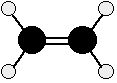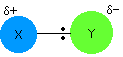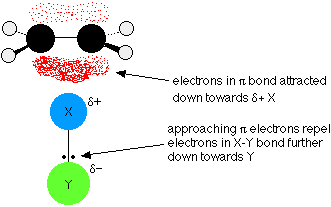# 8.1: Electrophilic Addition Reactions (EARs)

$$\newcommand{\vecs}{\overset { \rightharpoonup} {\mathbf{#1}} }$$ $$\newcommand{\vecd}{\overset{-\!-\!\rightharpoonup}{\vphantom{a}\smash {#1}}}$$$$\newcommand{\id}{\mathrm{id}}$$ $$\newcommand{\Span}{\mathrm{span}}$$ $$\newcommand{\kernel}{\mathrm{null}\,}$$ $$\newcommand{\range}{\mathrm{range}\,}$$ $$\newcommand{\RealPart}{\mathrm{Re}}$$ $$\newcommand{\ImaginaryPart}{\mathrm{Im}}$$ $$\newcommand{\Argument}{\mathrm{Arg}}$$ $$\newcommand{\norm}{\| #1 \|}$$ $$\newcommand{\inner}{\langle #1, #2 \rangle}$$ $$\newcommand{\Span}{\mathrm{span}}$$ $$\newcommand{\id}{\mathrm{id}}$$ $$\newcommand{\Span}{\mathrm{span}}$$ $$\newcommand{\kernel}{\mathrm{null}\,}$$ $$\newcommand{\range}{\mathrm{range}\,}$$ $$\newcommand{\RealPart}{\mathrm{Re}}$$ $$\newcommand{\ImaginaryPart}{\mathrm{Im}}$$ $$\newcommand{\Argument}{\mathrm{Arg}}$$ $$\newcommand{\norm}{\| #1 \|}$$ $$\newcommand{\inner}{\langle #1, #2 \rangle}$$ $$\newcommand{\Span}{\mathrm{span}}$$$$\newcommand{\AA}{\unicode[.8,0]{x212B}}$$

Learning Objective

• draw the general Electrophilic Addition Reaction (EAR) mechanism for an alkene

## Introduction

We are going to start by looking at ethene, because it is the simplest molecule containing a carbon-carbon double bond. What is true of C=C in ethene will be equally true of C=C in more complicated alkenes.Ethene , C2H4, is often modeled as shown above. The double bond between the carbon atoms is, of course, two pairs of shared electrons. What the diagram doesn't show is that the two pairs aren't the same as each other. One of the pairs of electrons is held on the line between the two carbon nuclei as you would expect, but the other is held in a molecular orbital above and below the plane of the molecule. A molecular orbital is a region of space within the molecule where there is a high probability of finding a particular pair of electrons.

In this diagram, the line between the two carbon atoms represents a normal sigma bond - the pair of shared electrons lies in a molecular orbital on the line between the two nuclei where you would expect them to be. The other pair of electrons is found somewhere in the shaded part above and below the plane of the molecule. This bond is called a pi bond. The electrons in the pi bond are free to move around anywhere in this shaded region and can move freely from one half to the other. The pi electrons are not as fully under the control of the carbon nuclei as the electrons in the sigma bond. Because the pi bond electrons lie exposed above and below the rest of the molecule, they are relatively open to reaction with other compounds.

In a sense, the pi bond is an unnecessary bond. The structure would hold together perfectly well with a single bond rather than a double bond. The pi bond often breaks and the electrons in it are used to join other atoms (or groups of atoms) onto the alkene molecule. To continue with our example, ethene undergoes addition reactions. An addition reaction is a reaction in which two molecules join together to make a bigger one. Nothing is lost in the process. All the atoms in the original molecules are found in the bigger one. The pi bond is electron rich and takes the role of the nucleophile seeking out an electrophile with its full or partial positive charge.

Using a general molecule X-Y as the electrophile reacting with ethene, the atoms 'X' and 'Y' are added to the carbon chain across the vinylic carbons as shown below.### Understanding the Electrophilic Addition Mechanism

The mechanism for the reaction between ethene and a molecule X-Y begins by recognizing the electrophilic nature of X-Y. Trends in relative electronegativity help identify bonds to create partial positives within polar compounds. As shown below, we are going to assume that Y is more electronegative than X, so that the pair of electrons is pulled slightly towards the Y end of the bond. This polarity means that the X atom carries a slight positive charge (partial positive).The slightly positive X atom is an electrophile and attracts the exposed pi electrons in the ethene. Now imagine what happens as they approach each other. The electrons in the half of the pi bond nearest the XY are attracted to the partial positive charge as shown below.The two electrons in the pi bond move closer towards the X until a covalent bond is made. Simultaneously, the electrons in the X-Y bond are pushed entirely onto the Y to form the anion Y- ion and a carbocation as shown below.### In the final step, the lone pair on Y- is attracted to the positively charged carbocation and they react to form a second covalent bond and the product below.### EAR Mechanism

The movements of the electrons for the EAR mechanism are shown with curved arrows.### Regiochemistry and Regioselective Reactions

Regiochemistry is the orientation of the electrophile relative to the pi bond of the alkene. Electrophilic addition reactions of alkenes can be regioselective depending on the symmetry and structure of the alkene. The details will be discussed in a later sections of this chapter.

Stereoselective Reactions

Different stereoisomeric reactants produce different stereoisomeric products. Electrophilic addition reactions of alkenes can be stereoselective depending on the symmetry and structure of the alkene. The details will be discussed in a later sections of this chapter.

Exercise $$\PageIndex{1}$$

1. In the next section, we will apply the Electrophilic Addition mechanism to actual compounds. Draw the complete mechanism when hydrogen bromide gas is bubbled through a solution of cyclopentene.

1.